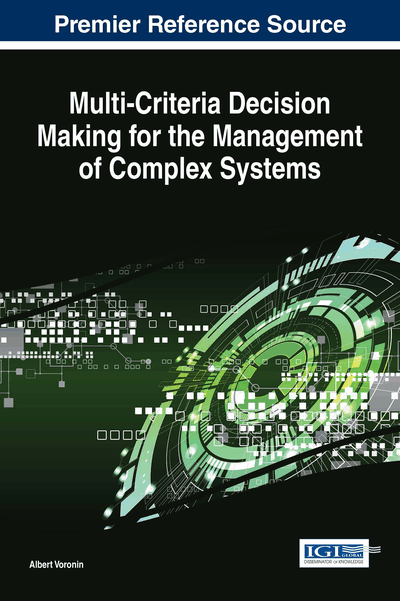# Multicriteria Optimization of Dynamic Control Systems

DOI: 10.4018/978-1-5225-2509-7.ch003
Available
\$37.50
No Current Special Offers

## Abstract

In Chapter 3, the problem and special assumptions to the problem are considered. Method of solving “Basic” problem and discussion of the method are given. Schemes of compromises are reviewed. Nonlinear scheme of compromises with respect to dynamic systems is proposed. Discussion of nonlinear scheme is given.
Chapter Preview
Top

## Introduction

In the previous Chapter, the concept of non-linear compromise scheme is introduced. This concept has specific features in the case of dynamic control systems.

Typically, complex control systems are dynamic systems. The term “dynamic system” was first introduced by Poincaré and meant any object which state changes with time. The behavior of a dynamic object in time is described by a system of differential equations, and the quality criterion is a functional defined on its solutions. Functional extremalization is the subject of the calculus of variations. In a multicriteria case difficulties of solving variational problems are repeatedly compounded due to the necessity of vector functionals extremization.

Optimal control theory organizes the transition process of a representative point in the phase space of a dynamic object from a starting point (area) to a given point (area) along such a trajectory that a functional, composed of the quality criteria of the process, gained extreme value. Found by solving the variational problem a multicriteria trajectory (an extremal) is called the program trajectory. If the representative point will move along the program trajectory, then the control process is optimal in the sense of a given vector criterion.

This movement is not stable with respect to arbitrarily small perturbations of both the boundary conditions and the external influences. Therefore, determining a program trajectory, we must then formulate the problem of synthesis of feedback laws guaranteeing stability to the movement along the found optimal trajectory. Thus, the modern optimal control theory involves the formulation and solution of two variational problems: 1) the problem of programming optimal trajectories, and 2) the task of synthesizing feedback laws that will lead to a steady movement of the object along the found extremals. In this work we consider the first of these tasks.

In the synthesis of complex modern dynamic systems, account has to be taken of widely different, often contradictory, requirements to the system performance; i.e., we urgently need to develop a formalized approach to the solution of multicriteria variational problems.

### The Problem

Given the system of differential equations describing the behavior of the control objectf(x,u,xg,z,t)=f(⋅), (1)

where x=x(t) is the state vector, u=u(t) is the control vector, xg=xg(t) is the vector of preassigned signals, x0=x(t0) is the vector of initial conditions, xf=x(T) is the final state vector, z=z(t) is the vector of disturbing forces, t∈[t0,T] is time, and f is the vector function of generalized force.

The external conditions xg, x0, xf, and z are specified in some form.

Let the given set of partial performance criteria of control system (1) form the vector

I=(Ii)i=1n. (2)

Each partial criterion Ii=Ii(⋅), i∈[1,n] is a functional, defined on the solutions of system of differential equations (1) with a control from the class of admissible controls U. The vector of partial criteria is restricted to an admissible domain

IM(I). (3)

The multicriteria variation problem amounts to finding the extremals{x*(t),u*(t)}, t∈[t0,T], u*∈U,I*∈M(I), (4) for which vector functional (2) is optimized. For a practical solution of the problem, special supplementary assumptions need to be made.

## Complete Chapter List

Search this Book:
Reset